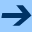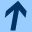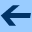Number representations

# Number representations

Let's have another look at my Collatz code. Remember that the conjecture was that the Collatz sequence always ends at one. We test this with some larger numbers:

$ktc >>> :load collatz3.kts >>> findMax(100000) Starting at 77031 needs 350 steps. >>> findMax(110000) Starting at 106239 needs 353 steps. >>> findMax(113000) Starting at 106239 needs 353 steps. >>> findMax(114000)  And at this point the program seems to go into an infinite loop! But that means that we do not reach the number one—did we manage to find a counter example to the Collatz conjecture? Let's try to find the starting value that leads to the infinite sequence. Here is a new function to do that (collatz4.kts): fun collatzBounded(n0: Int, steps: Int): Int { var n = n0 var count = 0 while (n != 1 && count < steps) { n = next(n) count += 1 } return count } fun findLong(n: Int, steps: Int) { for (i in 2 .. n) { val count = collatzBounded(i, steps) if (count >= steps) { println("Starting at$i needs $count steps.") } } }  Let's try it: >>> :load collatz4.kts >>> findLong(114000, 1000) Starting at 113383 needs 1000 steps. >>> findLong(114000, 10000) Starting at 113383 needs 10000 steps. >>> findLong(114000, 100000) Starting at 113383 needs 100000 steps. >>> findLong(114000, 1000000) Starting at 113383 needs 1000000 steps.  It seems that starting with 113383 leads to an infinite sequence! Let's print out the first numbers in this sequence. I add a print statement to collatzBounded (collatz5.kts): fun collatzBounded(n0: Int, steps: Int): Int { var n = n0 var count = 0 while (n != 1 && count < steps) { print("$n ")
n = next(n)
count += 1
}
println()
return count
}

The output is this:
>>> collatzBounded(113383, 200)
113383 340150 170075 510226 255113 765340 382670 191335 574006 287003
861010 430505 1291516 645758 322879 968638 484319 1452958 726479
2179438 1089719 3269158 1634579 4903738 2451869 7355608 3677804
1838902 919451 2758354 1379177 4137532 2068766 1034383 3103150 1551575
4654726 2327363 6982090 3491045 10473136 5236568 2618284 1309142
654571 1963714 981857 2945572 1472786 736393 2209180 1104590 552295
1656886 828443 2485330 1242665 3727996 1863998 931999 2795998 1397999
4193998 2096999 6290998 3145499 9436498 4718249 14154748 7077374
3538687 10616062 5308031 15924094 7962047 23886142 11943071 35829214
17914607 53743822 26871911 80615734 40307867 120923602 60461801
181385404 90692702 45346351 136039054 68019527 204058582 102029291
306087874 153043937 459131812 229565906 114782953 344348860 172174430
86087215 258261646 129130823 387392470 193696235 581088706 290544353
871633060 435816530 217908265 653724796 326862398 163431199 490293598
245146799 735440398 367720199 1103160598 551580299 1654740898
827370449 -1812855948 -906427974 -453213987 -1359641960 -679820980
-339910490 -169955245 -509865734 -254932867 -764798600 -382399300
-191199650 -95599825 -286799474 -143399737 -430199210 -215099605
-645298814 -322649407 -967948220 -483974110 -241987055 -725961164
-362980582 -181490291 -544470872 -272235436 -136117718 -68058859
-204176576 -102088288 -51044144 -25522072 -12761036 -6380518 -3190259
-9570776 -4785388 -2392694 -1196347 -3589040 -1794520 -897260 -448630
-224315 -672944 -336472 -168236 -84118 -42059 -126176 -63088 -31544
-15772 -7886 -3943 -11828 -5914 -2957 -8870 -4435 -13304 -6652 -3326
-1663 -4988 -2494 -1247 -3740 -1870 -935 -2804 -1402 -701 -2102 -1051
-3152 -1576 -788 -394 200

What's that? Why are there negative numbers? The last positive number is 827370449, so let's see what happens to that:
>>> next(827370449)
-1812855948

Oops! Is our definition of the next function wrong?
>>> 827370449 * 3
-1812855949

No, it seems that Kotlin's arithmetic is broken!

It turns out that you have to be careful with Int integer objects. Int integers have 32 bits, and therefore the largest possible Int value is $$2^{31} - 1$$. You can also find this maximum value as Int.MAX_VALUE. But $$3 \cdot 827370449$$ is larger than this. We can check this by computing the value using long arithmetic. A Long is an integer with 64 bits. You can convert an integer to a Long using the toLong() method, or simply write an L after a literal integer:

>>> next(827370449)
-1812855948
>>> 827370449 * 3
-1812855949
>>> Int.MAX_VALUE
2147483647
>>> 827370449 * 3
-1812855949
>>> 827370449.toLong() * 3
2482111347
>>> 827370449L * 3
2482111347
>>> Int.MAX_VALUE
2147483647
>>> Long.MAX_VALUE
9223372036854775807


#### How numbers are represented

Integers are represented using a fixed number of bits, as follows:

 Long 64 bits Int 32 bits Short 16 bits Byte 8 bits

Arithmetic on these types is performed by the hardware using registers of fixed length. For instance, imagine we had a type of integer with four bits, and we perform some additions:

 0010 = 2
+0111 = 7
---------
1001 = 9

1001 = 9
+0111 = 7
---------
0000 = 0

Since the register has only four bits, the overflow that happens when you add $$9 + 7$$ cannot be represented, and the result is not $$10000 = 16$$ but $$0000 = 0$$.

In other words, integer addition and subtraction is really addition and subtraction modulo $$2^{k}$$, where $$k$$ is the number of bits. For our four-bit data type, additions are modulo 16, and therefore $$9 + 7 = 0$$.

This is actually convenient, because it allows us to use negative numbers without any extra hardware: For instance, since $$-1 \equiv 15$$ (modulo 16), we can subtract one by adding $$15$$:

 0101 = 5
+1111 = 15 = -1
---------------
0100 = 4

0111 = 7
+1100 = 12 = -4
---------------
0011 = 3

Of course the problem is how to determine what number the output represents. When the result is $$1111$$, does that mean $$15$$ or $$-1$$?

The standard convention is to say that the number is negative when the first bit is one. In other words, $$1000 ... 1111$$ are $$-8$$ to $$-1$$, and $$0000 ... 0111$$ are $$0$$ to $$7$$:

0111 = 7
0110 = 6
0101 = 5
0100 = 4
0011 = 3
0010 = 2
0001 = 1
0000 = 0
1111 = -1
1110 = -2
1101 = -3
1100 = -4
1011 = -5
1010 = -6
1001 = -7
1000 = -8

This is convenient, because it's very easy to test if a number is negative, but it's just a convention. Some programming languages (like C and C++) also have unsigned integers, where $$0000 ... 1111$$ mean $$0$$ to $$15$$. In principle, we could also say that $$1100 ... 1111$$ mean $$-4$$ to $$-1$$, and $$0000 ... 1011$$ mean $$0$$ to $$11$$.

In our example above, we performed the multplication $$3 * 827370449 = 2482111347$$. In binary, this is

11 * 00110001010100001010101111010001 =
10010011111100100000001101110011

The first bit of the result is $$1$$, and therefore it is considered negative.

You can learn more about number representations here.Number representations